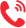更多 >>

学校：
地区：

天津大学
• 专业名称
• 所属学院
• 学制
• 学费(元)
• 地区
• 简章详情
• 报名
大连理工大学
• 专业名称
• 所属学院
• 学制
• 学费(元)
• 地区
• 简章详情
• 报名
同济大学
• 专业名称
• 所属学院
• 学制
• 学费(元)
• 地区
• 简章详情
• 报名
武汉大学
• 专业名称
• 所属学院
• 学制
• 学费(元)
• 地区
• 简章详情
• 报名
华中科技大学
• 专业名称
• 所属学院
• 学制
• 学费(元)
• 地区
• 简章详情
• 报名
四川大学
• 专业名称
• 所属学院
• 学制
• 学费(元)
• 地区
• 简章详情
• 报名
太原理工大学
• 专业名称
• 所属学院
• 学制
• 学费(元)
• 地区
• 简章详情
• 报名
中国矿业大学
• 专业名称
• 所属学院
• 学制
• 学费(元)
• 地区
• 简章详情
• 报名
合肥工业大学
• 专业名称
• 所属学院
• 学制
• 学费(元)
• 地区
• 简章详情
• 报名
福州大学
• 专业名称
• 所属学院
• 学制
• 学费(元)
• 地区
• 简章详情
• 报名
中国地质大学(武汉)
• 专业名称
• 所属学院
• 学制
• 学费(元)
• 地区
• 简章详情
• 报名
四川农业大学
• 专业名称
• 所属学院
• 学制
• 学费(元)
• 地区
• 简章详情
• 报名
中国矿业大学(北京)
• 专业名称
• 所属学院
• 学制
• 学费(元)
• 地区
• 简章详情
• 报名
中国地质大学(北京)
• 专业名称
• 所属学院
• 学制
• 学费(元)
• 地区
• 简章详情
• 报名
河北大学
• 专业名称
• 所属学院
• 学制
• 学费(元)
• 地区
• 简章详情
• 报名
华北水利水电大学
• 专业名称
• 所属学院
• 学制
• 学费(元)
• 地区
• 简章详情
• 报名
中北大学
• 专业名称
• 所属学院
• 学制
• 学费(元)
• 地区
• 简章详情
• 报名
辽宁工程技术大学
• 专业名称
• 所属学院
• 学制
• 学费(元)
• 地区
• 简章详情
• 报名
吉林建筑大学
• 专业名称
• 所属学院
• 学制
• 学费(元)
• 地区
• 简章详情
• 报名
苏州科技大学
• 专业名称
• 所属学院
• 学制
• 学费(元)
• 地区
• 简章详情
• 报名
浙江工业大学
• 专业名称
• 所属学院
• 学制
• 学费(元)
• 地区
• 简章详情
• 报名
山东科技大学
• 专业名称
• 所属学院
• 学制
• 学费(元)
• 地区
• 简章详情
• 报名
河南大学
• 专业名称
• 所属学院
• 学制
• 学费(元)
• 地区
• 简章详情
• 报名
武汉科技大学
• 专业名称
• 所属学院
• 学制
• 学费(元)
• 地区
• 简章详情
• 报名
西安理工大学
• 专业名称
• 所属学院
• 学制
• 学费(元)
• 地区
• 简章详情
• 报名
兰州理工大学
• 专业名称
• 所属学院
• 学制
• 学费(元)
• 地区
• 简章详情
• 报名

东华理工大学
• 专业名称
• 所属学院
• 学制
• 学费(元)
• 地区
• 简章详情
• 报名

更多 >>

更多 >>

## 联系方式400-101-7010

报名咨询热线

400-101-7010